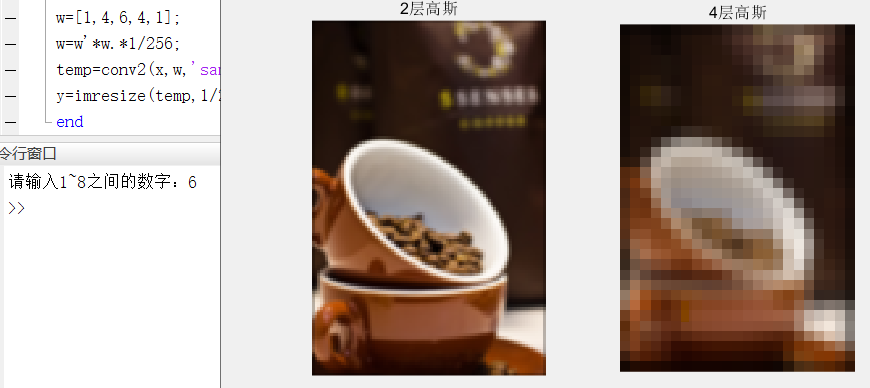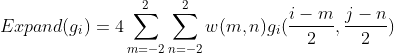• matlab高斯金字塔代码图像拼接 这是来自的项目。图像拼接是将一系列具有重叠字段的图像组合成诸如全景图的混合结果的过程。 该实现包括通过SIFT描述符进行特征点检测，通过KNN（来自）进行图像匹配，最后通过alpha...
• 生成高斯或拉普拉斯金字塔，或从金字塔重建图像。 包含使用金字塔进行图像混合的演示脚本。 该函数比Matlab函数impyramid使用更方便。matlab
• 图像金字塔算法matlab

热门讨论 2010-04-14 15:53:49
图像高斯金字塔、拉普拉斯金字塔的下采样，通过matlab实现
• 亲测可用 （1）matlab平台 （2）高斯金字塔 （3）生成金字塔第五层
• 这个包包含一些用于多尺度图像MatLab 工具加工。 简而言之，这些工具包括： - 递归多尺度图像分解（金字塔），包括拉普拉斯金字塔、QMF、小波和可控金字塔。 这些对任意维度的一维或二维信号进行操作。 数据结构...matlab
• 高斯图像金字塔        有没有使用过PS软件的小伙伴呀，里面有一项模糊的功能就叫做高斯模糊，用高斯模糊就可以生成‘马赛克’区域。本次要实现的高斯金字塔的原理就和PS的高斯...

高斯图像金字塔

有没有使用过PS软件的小伙伴呀，里面有一项模糊的功能就叫做高斯模糊，用高斯模糊就可以生成‘马赛克’区域。本次要实现的高斯金字塔的原理就和PS的高斯模糊原理是相同的。
原理
在高斯金字塔中，最重要的就是高斯核。我们需要使用高斯核对原图像做卷积处理。在这之后，进行一次原图大小二分之一的下采样，这样就生成了一层高斯图像。高层的高斯图像对底层的图像做上述操作即可。
高斯核： 将其理解成一个权值就可以了，使用高斯核提供的权值对原像素进行加权的操作。
卷积： 卷积(英语：Convolution)是通过两个函数f 和g 生成第三个函数的一种数学算子，以本文为例，就是用像素点+高斯核=新像素点的操作步骤。
高斯核：步骤
①、构造高斯卷积核。
②、进行卷积操作。
③、进行下采样操作。
④、建立一个cell存入每一层的高斯图像。
⑤、对新生成的图像重复②~④步骤。
⑥、最终cell数组中存放了高斯金字塔。

MATLAB实现

clear;clc;

R=I(:,:,1);G=I(:,:,2);B=I(:,:,3);                %取出R,G,B三个通道。

x=input('请输入1~8之间的数字：');                 %依据图像大小设定高斯层数。
for i=1:x
R=fgaosi(R);G=fgaosi(G);B=fgaosi(B);
y{i}=cat(3,R,G,B);                           %将三个通道拼接回RGB彩色图像。
end
subplot(1,2,1);imshow(uint8(y{2}));title('2层高斯')
subplot(1,2,2);imshow(uint8(y{4}));title('4层高斯')

function y=fgaosi(x)
w=[1,4,6,4,1];
w=w'*w.*1/256;                                   %构造卷积核
temp=conv2(x,w,'same'); %help conv2              %进行卷积操作
y=imresize(temp,1/2);                            %进行下采样操作
end

运行结果：关于conv2卷积操作的使用方法，在系统中有写，很详细。关于高斯核的构造和使用，根据实际需求不同，也可以对它做出修改，其本质也是值得思考和琢磨。高斯金字塔对于实现拉普拉斯金字塔是必要的。因为后者是前者的差值金字塔。当然，直接采用高斯金字塔也可以实现图像融合。

展开全文matlab 计算机视觉
• 方法1：先高斯滤波，再降采样 im = imread( 'image_...方法2：直接利用matlab提供的video.Pyramid类实现 pyr = video.Pyramid; pyr.PyramidLevel = 3; im3 = step( pyr, im ); figure, imshow(mat2gray(double(im3)));

方法1：先高斯滤波，再降采样

if ndims( im ) == 3

im = rgb2gray( im );

end

% generate a Gaussian kernel

ker = fspecial( 'gaussian', 3, 0.5 );

im = imfilter( im, ker, 'conv', 'symmetric', 'same' );

[im_h, im_w] = size( im );

% image down sampling

im2 = im( 1 : 2 : im_h, 1 : 2 : im_w );

figure, imshow( im ); title( 'original image' );

figure, imshow( im2 ); title( 'resampled image' );方法2：直接利用matlab提供的video.Pyramid类实现

pyr = video.Pyramid;

pyr.PyramidLevel = 3;

im3 = step( pyr, im );

figure, imshow(mat2gray(double(im3)));

展开全文• 图像金字塔

2021-04-21 01:15:02
图像金字塔图像处理和计算机视觉中常用到的概念，常常用于多尺度处理领域(multiscale processing)，尤其早年的图像匹配、识别等算法中都用到了...高斯金字塔我们可以使用如下Matlab代码来进行得到高斯金字塔：func...

图像金字塔是图像处理和计算机视觉中常用到的概念，常常用于多尺度处理领域(multiscale processing)，尤其早年的图像匹配、识别等算法中都用到了图像金字塔。

高斯金字塔(Gaussian pyramid)

下图为高斯金字塔的示意图，金字塔的底层为原始图像，每向上一层则是通过高斯滤波和1/2采样得到(去掉偶数行和列)。高斯金字塔

我们可以使用如下Matlab代码来进行得到高斯金字塔：

function [ pyr ] = gaussian_pyramid( I,nlev )

%GAUSSIAN_PYRAMID Summary of this function goes here

% Detailed explanation goes here

pyr = cell(nlev,1);

pyr{1} = I;

filter = fspecial('gaussian');

for i=2:nlev

% gaussian filter

I = imfilter(I,filter,'symmetric');

% downsample

I = I(1:2:end,1:2:end);

pyr{i} = I;

end

end

下图就是生成的金字塔图像金字塔图像

高斯滤波器可以看做一个低通滤波器，那么每经过一次的高斯滤波，图像中仅能够保留某个频率值以下的频率部分，所以高斯金字塔也可以看做一个低通金字塔(每一级只保留某个频率以下的成分)。

拉普拉斯金字塔(Laplacian pyramid)

在进行高斯金字塔运算时，由于不断的进行高斯滤波和下采样，我们丢失了很多高频信号，而拉普拉斯金字塔的目的就是保存这些高频信号，保存这些高频信号所采用的方式就是保存差分图像。比如，拉普拉斯金字塔的第０层，就是原始图像和原始图像下采样(Reduce)后再次上采样(Expand)的图像的差值。

function pyr = laplacian_pyramid(I,nlev)

r = size(I,1);

c = size(I,2);

if ~exist('nlev')

% compute the highest possible pyramid

nlev = floor(log(min(r,c)) / log(2));

end

% recursively build pyramid

pyr = cell(nlev,1);

filter = pyramid_filter;

J = I;

for l = 1:nlev - 1

% apply low pass filter, and downsample

I = downsample(J,filter);

odd = 2*size(I) - size(J); % for each dimension, check if the upsampled version has to be odd

% in each level, store difference between image and upsampled low pass version

pyr{l} = J - upsample(I,odd,filter);

J = I; % continue with low pass image

end

pyr{nlev} = J; % the coarest level contains the residual low pass image

%下采样函数

function R = downsample(I, filter)

border_mode = 'symmetric';

% low pass, convolve with separable filter

R = imfilter(I,filter,border_mode); %horizontal

R = imfilter(R,filter',border_mode); %vertical

% decimate

r = size(I,1);

c = size(I,2);

R = R(1:2:r, 1:2:c, :);

％上采样函数

function R = upsample(I,odd,filter)

% increase resolution

I = padarray(I,[1 1 0],'replicate'); % pad the image with a 1-pixel border

r = 2*size(I,1);

c = 2*size(I,2);

k = size(I,3);

R = zeros(r,c,k);

R(1:2:r, 1:2:c, :) = 4*double(I); % increase size 2 times; the padding is now 2 pixels wide，注意这里要乘以４！

% interpolate, convolve with separable filter

R = imfilter(R,filter); %horizontal

R = imfilter(R,filter'); %vertical

% remove the border

R = R(3:r - 2 - odd(1), 3:c - 2 - odd(2), :);

％产生拉普拉斯滤波器

function f = pyramid_filter()

f = [.05, .25, .4, .25, .05]; % original [Burt and Adelson, 1983]

%f = [.0625, .25, .375, .25, .0625]; % binom-5

f = f'*f;

end

通过上面的代码，我们可以得到拉普拉斯金字塔如下所示。拉普拉斯金字塔

由于拉普拉斯金字塔保留了高频信号，那么我们可以用它来重建原始图像。

function R = reconstruct_laplacian_pyramid(pyr)

r = size(pyr{1},1);

c = size(pyr{1},2);

nlev = length(pyr);

R = pyr{nlev};

filter = pyramid_filter;

for l = nlev - 1 : -1 : 1

% upsample, and add to current level

odd = 2*size(R) - size(pyr{l});

R = pyr{l} + upsample(R,odd,filter);

%figure

%imshow(R,[]);

%imwrite(mat2gray(R),[num2str(l),'.jpg']);

end重建的图像

需要注意的地方

为什么在处理高斯金字塔的时候需要采用滤波呢，直接下采样不可以吗？

如果把图像看做频率信号的话，直接进行下采样则会出现采样不足的情况，消除这种情况的方法是采用低通滤波器(高斯滤波器)对图像进行滤波，将采样不足的高频信号过滤掉，这样在进行下采样的时候就保证了不出现采样不足的情况。

一般在图像处理中，将上面Matlab实现的下采样函数(包括高斯滤波和图像尺寸减半)这一部分叫做Reduce，将上面Matlab实现的上采样函数(包括高斯滤波和图像尺寸增加一倍)这部分叫做Expand，如果用数学方式表达的话，Expand函数如下：注意前面需要乘以４。

拉普拉斯金字塔可以看做一个带通滤波器，在每一级都保留了图像某个频率值附近的成分。(这一点与高斯金字塔不同，高斯金字塔是低通金字塔)

两个低通滤波器的差值就构成了一个带通滤波器。两个低通之差，构成带通滤波器

Python实现

下面是高斯金字塔和拉普拉斯金字塔的Opencv-Python实现

import cv2

import numpy as np

def gaussian_pyr(img,lev):

img = img.astype(np.float)

g_pyr = [img]

cur_g = img;

for index in range(lev):

cur_g = cv2.pyrDown(cur_g)

g_pyr.append(cur_g)

return g_pyr

def laplacian_pyr(img,lev):

img = img.astype(np.float)

g_pyr = gaussian_pyr(img,lev)

l_pyr = []

for index in range(lev):

cur_g = g_pyr[index]

next_g = cv2.pyrUp(g_pyr[index+1])

cur_l = cv2.subtract(cur_g,next_g)

l_pyr.append(cur_l)

l_pyr.append(g_pyr[-1])

return l_pyr

def lpyr_recons(l_pyr):

lev = len(l_pyr)

cur_l = l_pyr[-1]

for index in range(lev-2,-1,-1):

print(index)

cur_l = cv2.pyrUp(cur_l)

next_l = l_pyr[index]

cur_l = cur_l + next_l

return cur_l

展开全文• matlab高斯金字塔代码拉普拉斯金字塔 过滤器的GPU实现 该库包括过滤器和拉普拉斯金字塔的GPU实现。 还在openCV中实现了本地拉普拉斯金字塔和混合示例。 与此处给出的原始MATLAB代码相比，GPU代码可以非常快速地运行...
• matlab impyramid图像金字塔

千次阅读 2018-10-15 13:13:03
B = impyramid（I, direction） direction 可取‘reduce’或‘expand’，表示图像的宽度与高度缩小为原来的1/2与变大为原来的2倍

B = impyramidI, direction

direction 可取‘reduce’或‘expand’，表示图像的宽度与高度缩小为原来的1/2与变大为原来的2倍

展开全文• MATLAB GUI实现图像Laplace金字塔分解与融合，能够通过两幅或多幅图像的融合处理，得到细节丰富、灰度分布均匀的图像Matlab
• 这包含用于高斯图像金字塔的“扩展”和“减少”例程的代码，如论文中所述 - “ 拉普拉斯金字塔作为紧凑图像代码” - Burt 和 Adelson，1983 年。 其重要的应用包括运动估计。matlab
• 该楼层疑似违规已被系统折叠隐藏此楼查看此楼function [gauss_pyr] = build_gauss_pyr(base, octvs,intvls,sigma)sig = zeros(1,intvls+3);gauss_pyr = cell(octvs,intvls+3);sig(1) = sigma;k = 2^(1/intvls);...
• 该压缩包内含有基于梯度金字塔变换的多模态图像融合方法的MATLAB代码，可以直接运行，得到融合结果。另外，压缩包内包括了多组已配准、待融合的图像示例，具体包括红外图像与可见光图像、CT与MR图像，多聚焦图像。...
• 一、简介 二、源代码 clear all; close all; clc; im1 = (imread('红外.jpg'));...im2 = imread('可见光.jpg');...if length(size(im1)) ==3%判断输入图像是否是真彩色图像 im1 = rgb2gray(im1); end if length(size(imatlab 计算机视觉 opencv
• 1.1 图像多分辨率金字塔高斯金字塔和拉普拉斯金字塔。%生成高斯滤波器的核w=fspecial('gaussian',3,0.5)%进行高斯滤波g=imfilter(I,w,'conv','symmetric','same') 多分辨率金字塔化一般包括两个步骤：低通滤波...
• Matlab代码金字塔学习用于人姿估计的特征金字塔 本文的培训和测试代码 杨伟，李爽，欧阳万里，李洪生，王小刚ICCV，2017年 此代码基于和。 感谢作者。 安装 安装 。 安装依赖项。 luarocks install hdf5 luarocks ...
• 高斯金字塔的matlab实现

热门讨论 2011-08-16 09:46:19
图像高斯金字塔matlab实现，主要分为高斯平滑与向下采样两部分，可直接运行高斯金字塔
• matlab高斯金字塔代码要求 由Vidrio Technologies。 版本≥5.2.4（） 其他版本，包括非免费版本，也可以使用，但未经测试。 PrintImage要求在ScanImage中配置以下硬件： 共振扫描仪 快速Z压电平台 由快速模拟量输出...
• 写在前面在文章《特征，特征不变性，尺度空间与图像金字塔》中我们初步谈到了图像金字塔，在这篇文章中将介绍如何在人脸检测任务中构建输入图像金子塔。人脸检测中的图像金字塔人脸检测任务，输入是一张图像，输出...
• Matlab代码金字塔DCPDN 密集连接的金字塔除雾网络（CVPR'2018） ， []（CVPR'18） 我们提出了一种新的端到端单图像除雾方法，称为密集连接金字塔除雾网络（DCPDN），该方法可以共同学习透射图，大气光和除雾。 通过...
• 1、高斯金字塔计算步骤 （1）对第 iii 层图像进行高斯内核卷积； （2）将所有偶数行和列去除（下采样），得到第 i+1i+1i+1 层图像；...（3）与高斯金字塔第同层次图像相减，就是图像在缩小和放大整个过程中减少的信.
• 先上代码 function [r,c,nccImg] = pyramidMatch...% 过程：(1)为待匹配的图像和模板图像创建nLevels层金字塔图像 % (2)从金字塔最高层开始进行匹配，最高层的要完全扫描匹配，得到的最佳匹配位置 % 后向下层传递matlab 模板匹配
• clearinputPath ='D:\数字图像处理\研究方向\Filter Smooth\images\';filename = [inputPath 'pai.png'];I = im2double(imread(filename));if size(I,3)==3I=rgb2gray(I);endnlev=4;sigma=1;sigma_r=0.1;f = [.05, ....
• Matlab图像融合】高斯金字塔+拉普拉斯金字塔彩色图像融合【含GUI源码 1506期】 二、matlab版本及参考文献 1 matlab版本 2014a 2 参考文献  蔡利梅.MATLAB图像处理——理论、算法与实例分析[M].清华大学出版社，...matlab 图像处理 算法
• 高斯金字塔可以作为 图像特征提取 的预处理步骤—————————————————————————————————————————————————————————————————————————————...
• 对源图像进行融合时,首先对图像进行分解,利用拉普拉斯金字塔分解,先对图像进行高斯金字塔分解,然后再进行拉普拉斯金字塔分解。 1 高斯金字塔分解 记源图像为G0,G0即为高斯金字塔最底层,将其进行高斯低通滤波,之后对...matlab 计算机视觉 开发语言
• 我使用了 "imfilter" ，它是图像处理工具箱的一部分。 如果您没有图像处理工具箱，请将“imfilter”更改为“filter2”，该功能应该可以正常工作。matlab
• 一、高斯-拉普拉斯金字塔 简介        第6节的内容是高斯金字塔，拉普拉斯金字塔还称为高斯-拉普拉斯金字塔。是因为它的生成方式如下：       &...matlab 计算机视觉...

matlab图像金字塔matlab 订阅